# BSCI 1510L Literature and Stats Guide: 3.1 Describing Quantities and Their Variation

Introduction to Biological Sciences lab, first semester

## 3.1 Describing Quantities and Their Variation

In order to assess and discuss variation in quantities, we need mathematical tools to describe the variation.  The variation in many (but not all) quantities has a distribution known as the normal distribution or "bell-shaped curve".  (In the exercises in this course, unless otherwise noted we will assume that the quantities that we are working with are normally distributed.)

## 3.1.1 The mean

When we say that we want to know the value of a quantity, what we usually really want to know is the value of the center of the distribution.  The most common way of describing this is the mean (also known as the average).  The method of calculating a mean is well-known.  If we sample and make repeated measurements of some quantity, Y, we can calculate the mean value of Y (signified by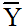):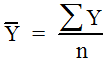Equation 1

whereis the sum of all of the Y values measured in the experiment and n is the total number of measurements.

The mean of a quantity gives us a feeling of the "size" of the quantity, but it tells us nothing about its variability.  The figures below show the simulated distributions of the masses of 100 wild M. musculus individuals and 100 laboratory-strain M. musculus individuals.  In this type of graph (called a histogram), the height of each bar represents the number of individuals included in the range defined by the width of the bar.Fig. 1.a. mass of wild mice (g)Fig. 1. b. mass of lab mice (g)

The mean masses of both mice populations are 25 grams.  However, you can see that the wild mice have much more variable masses, perhaps because they had a more variable environment, food supply, or genetic background than the lab mice.

## 3.1.2 The variance (s^2)

One commonly used measure of the variability of a quantity is called the variance (s2).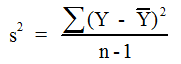Equation 2

The difference of each measurement of Y from the mean is squared: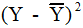, these differences are then summed: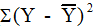and divided by the total number of measurements minus one (n-1).

## 3.1.3 The standard deviation (s)

A second measure of the variability is called the standard deviation (s):Equation 3

where s is the square root of the variance. Standard deviation is a more intuitive measure than variance for two reasons.  One is that the units of standard deviation are the same as the units of the values themselves.  Another reason is that in a normal distribution, 68% of samples fall in the range of one standard deviation above or below the mean.  For these reasons, we will generally use standard deviation rather than variance in our work.Fig. 2.a.  mass of wild mice (g)Fig. 2.b. mass of lab mice (g)

Returning to the example of the mice, the standard deviation of the wild mice is 7.3 gram and the standard deviation of the lab mice is 3.3 gram.  The range of +/- one standard deviation for the lab mice is 21.7g to 28.3g and it includes 68 of the 100 mice.  The range of +/- one standard deviation for the wild mice is 17.7g to 32.3g.  Thus one can see that the standard deviation provides a measure of the likely spread of measurements for each mouse population.